# Numerical Simulation of the Motion of Solid Particles in a Stirred Tank

Numerical Simulation of the Motion of Solid Particles in a Stirred Tank
Department of Mechanical Engineering, Shahrekord University, Shahrekord 88186-34141, Iran

Corresponding Author Email:
m.bayareh@sku.ac.ir
Page:
109-116
|
DOI:
https://doi.org/10.18280/ijht.370113
1 April 2018
|
Accepted:
21 December 2018
|
Published:
31 March 2019
| Citation

OPEN ACCESS

Abstract:

The motion of solid spherical particles in a baffled stirred tank is investigated numerically. The turbulent flow is simulated by using a two-phase model with the standard k-ε turbulence model. The standard k-ε turbulence model has been used to simulate single and multi-phase flows in stirred tanks. In the present work, this model is used to evaluate the liquid mixing time. A multiple reference frames (MRFs) method is employed to simulate the rotation of impeller in the tank. Eulerian-Eulerian and Eulerian-Lagrangian approaches are implemented to simulate a dense solid-liquid suspension. The particle size is 264 µm concludes the volume fraction of 10 %. Also, the impeller rotational speed is in the range of 5 to 16.7 rps. The results show that the mixing quality increases with the impeller rotational speed. It is revealed that the Eulerian-Eulerian approach that captures the solid-liquid interface is more appropriate model to predict the mixing process than Eulerian-Lagrangian one (point-particle method) for a dense suspension.

Keywords:

stirred tank, mixing, particle concentration, turbulent flow, Eulerian-Eulerian method, Eulerian-Lagrangian method

1. Introduction

Multiphase flows are widely observed in nature and various industries, such as oil and gas industries, chemical processes, and power plants. Suspension of a solid-liquid phase is one of the most important application of two-phase flows in chemical, biochemical, and mineral compound analysis. Particular examples of solid-liquid systems can be found in multiphasic catalyst transitions, crystallization, purification and deposition, decomposition, coagulation and flocculation processes, and water behaviors. The presence of suspended solid particles changes the flow pattern relative to the state in which these particles are not present. This change is due to the inertia of the particles, their interaction with vortices and turbulences, the fall of particles and the formation of the substrate, the presence of force in the coarser particles and the energy loss due to the effect of this force, and the effects of neighboring particles.

Several studies have been carried out on two-phase flows that were able to predict the behavior of solid-liquid particles for different operating conditions and geometries. Yamazaki et al. experimentally measured the concentration of solid partilces in a mixing tank and showed that the ratio of liquid fluid velocity and solid particle descending velocity are functions of rotational speed of the impeller . Gosmon et al. presented a model to simulate the turbulent two-phase flow in a mixing reactor . They found that maximum particle deposition occurs at the vicinity of the walls. Sbrizzai et al. simulated the dispersion of suspended inertial particles in an un-baffled stirred tank using an immersed-boundary method . Three suspension of 30, 50 and 100 micrometer diameter particles were considered. Their results showed that smaller particles (30 μm) remain randomly dispersed in the tank and larger particles accumulate at the bottom of the tank.

Solid-liquid synthetic reactors are widely used in the chemical industries. The performance of these reactors is controlled by the suspension suppression. Kasat et al. studied the turbulent liquid-solid flow in a stirred reactor and demonstrated that the mixing time increases with the angular velocity of the impeller . Radl and Sundaresan numerically presented a post-force model for simulating solid-gas suspensions based on the Euler- Lagrange view . In this study, the drag relation was corrected for particles in the fluid. Using the coarse grains of the fluid, the average velocity of the particles deposited was proportional to the particle size. Montante et al. measured the solid suspended particles inside a reservoir using a particle velocity image . They investigated the effect of the solid phase on the average velocity and the degree of continuous phase turbulence, as well as the local slip rate of solid-liquid particles. One of the most striking results in this work is the reduction of the amount of turbulence by decreasing the size of solid suspended particles. Bashiri et al. analyzed the turbulent fluid flows in agitator tanks using the radioactive particles tracking (RPT) technique . They demonstrated that the RPT approach leads to acceptable results for simulating turbulent flow along with the mixing process of stirred tanks. Lassaigne et al. experimentally investigated the mixture of viscous liquid and high-density particles in a synthetic repository . They evaluated the mixture of high viscous spherical particles by determining the suspension rate, homogeneous mixture speed, and the time to reach the homogeneous mixture. Their results showed that the suspension rate decreases with the diameter of the particles and the viscosity of the liquid. Wadnererk et al. simulated a solid-liquid diphosphorus mixture in a tank for low-density solid-liquid systems . Solid-liquid hydrodynamics plays a vital role in the design and operation of the mixing tank as a mixing system. Tamburini et al. considered dense solid-liquid suspension systems (of volume fraction 11.9 %) in baffled stirred tanks to explore the minimum value of the impeller rotational speed that guarantees the homogeneity of suspensions . They showed that MRFs and transient sliding grid methods lead to similar results approximately.

Recently, Oyegbile et al. investigated the characteristics of a rotating disk in a stirred tank numerically and experimentally . They found that the tangential velocity increases across the disk and kinetic energy enhances as the radial distance increases. Liu et al. studied the effect of inlet velocity on the motion of single and multiple particles in an impinging flow rector using realizable k-ε turbulence model . They reported three flow regimes for the case of multiple particles and demonstrated that these regimes causes the particles to oscillate or accelerate again in the tank.

There are different turbulence models to evaluate the turbulent viscosity. Although the standard k-ε turbulence model has been used to simulate these flows by many researchers, this model is not appropriate to estimate the turbulent quantities of rotating flows . To predict the behavior of solid suspension in these flows, large eddy simulation (LES) is employed. However, the LES requires very high computational time. In the present work, the standard k-ε turbulence model is used to calculate the liquid time mixing not to predict the turbulent quantity of the rotating flow. Hence, this turbulence model is appropriate for further simulations.

Main objective of the present work is that of simulating a dense solid-liquid suspension (of 10 % solid volume fraction) in a baffled stirred tank by using Eulerian-Eulerian and Eulerian-Lagrangian approaches along with standard k-ε turbulence model. A multiple reference frames (MRFs) method is used to model the impeller rotation. The present results are compared with the experimental results of Yamazaki et al. . Current predictions are used to explain the mixing process and to compare the effectiveness of two models.

2. Problem Setup and Numerical Method

In this paper, the interaction between solid particles in a stirred tank is investigated to explore the fluid mixing process characteristics. Eulerian-Eulerian and Eulerian-Lagrangian models are employed to simulate the motion of spherical solid particles in the tank.

The geometry of the problem consists of a motor and accessories, a power transfer shaft from the engine to the impellers in the tank and cylindrical tank. Due to the simplicity of the work, numerical simulations of the engine modeling are avoided .All dimensions of the tank, the properties of fluid and solid particles in the present study are the same as the experimental data used by Yamazaki . In addition, numerical results reported by Kasat et al. are used for comparison and validation . Hence, the diameter and the height of cylindrical reactor is H=30 cm and L=H, respectively. There are four attached baffles perpendicular to the tank wall (of width 0.1 H). A propeller and an impeller are integrated as parts of the reactor, which are responsible for driving power from the engine to the inner part of the tank and eventually the fluid. The propeller shaft is directed down the tank from the top of the tank. The length of the shaft is 90 mm and the diameter of the impeller is 70 mm. Solid particles (of average diameter, dp =264 µm and the density, $ρ_p$ =2470 kg/ m3 ) are suspended in water (of the density, $ρ_w$ =1000 kg/ m3  and the viscosity, $μ_w$ =0.001 kg/ms). The volume fraction of solid particles is 10 % for all simulations. Computational domain is illustrated in Figure 1. Half of the tank is considered for the simulations due to the flow symmetry.

Governing equations for solving the fluid flow and soluble particles are continuity and momentum equations. Since the propeller rotation in the tank is simulated by multiple reference frames method (MRFs), the governing equations include continuity and momentum equations are expressed as follows, respectively:

$∂ρ/∂t+∇.ρv ⃗_r=0$ (1)

$(∂ρv ⃗)/∂t+∇.(ρv ⃗_r v ⃗ )+ρ(ω ⃗×v ⃗ )=-∇P+ρg ⃗+∇.τ ̿+F ⃗$ (2)

where $ρ$ is the fluid density, $v ⃗_r$ is the velocity relative to the rotating coordinates, $v ⃗_r$ is the absolute velocity vector,  $ω ⃗$ is the angular velocity, P is the static pressure, $ρg ⃗$ is the gravitational force, $F ⃗$ is the external volumetric force, and $τ ̿$ is the shear stress tensor.

## 1.png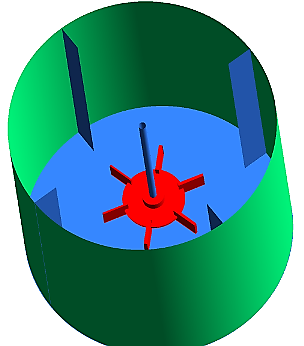Figure 1. Computational domain of stirred tank

The standard k-ε turbulence model is a semi-empirical model based on solving transfer equations for the turbulent kinetic energy and its loss rate. The equation k is obtained from the momentum equation. In order to obtain the equations k and ε, it is assumed that the flow is totally turbulent and the effects of the molecular viscosity are negligible. The transport equations for k and ε are as follows, respectively :

$\frac{∂(r_α ρ_α k)}{∂t}+∇(r_α ρ_α kv ⃗_α-r_α (μ_α+\frac{μ_t}{σ_k} )∇k)=r_α S_k$ (3)

$\frac{∂(r_α ρ_α ε)}{∂t}+∇(r_α ρ_α εv ⃗_α-r_α (μ_α+\frac{μ_t}{σ_ε} )∇ε)=r_α S_ε$ (4)

where the source terms for kinetic energy, k and turbulent dissipation rate, ε are as follows:

$S_k=μ_t∇v_α (∇v_α+(∇v_α )^T )-ρ_α ε$ (5)

$S_ε=C_1 \frac{ε}{k}μ_t ∇v_α (∇v_α+(∇v_α )^T )-C_2 ρ_α \frac{ε^2}{k}$ (6)

$μ_t=ρ_α C_μ \frac{k^2}{ε}$ (7)

where  is the turbulent viscosity and α indicates the phase. Also, the constant coefficients are: C1=1.44, C2=1.92, $σ_k=1$, $σ_ε=13$ and $C_μ=0.09$

For the Eulerian-Eulerian model, the basic form of the momentum transport equation for the mixture of solid and liquid particles is considered as follows:

$ρ\frac{∂v ⃗}{∂t}+ρ(v ⃗.∇) v ⃗=-∇P+ρg ⃗+∇.(μ(∇v ⃗+(∇v ⃗ )^T )-∇.(ρC_S (1-C_S)u ⃗_slip u ⃗_slip$(8)

where, Cs is mass fraction of particles and $u ⃗_slipis$  is the relative velocity between solid and liquid phases. For the density of the mixture, the following equation is used:

$ρ=(1-φ_S ) ρ_f+φ_S ρ_S$ (9)

where, $ρ_f$  and $ρ_s$  are the density of the liquid phase and solid particles, respectively. $φ_S$  is the volume fraction of the solid phase.

The governing equations are discretized by a control volume-based finite-difference method. The SIMPLE algorithm is used for coupling the velocity and the pressure. A no-slip wall boundary condition is employed at the solid surfaces. Also, zero shear stress boundary condition is used as a free surface boundary condition. The convergence criterion at each stage is smaller than 10-6 .

3. Results

3.1 Eulerian-Eulerian approach

The fluid dynamics of a baffled stirred tank is investigated in the present study. Initially four grid resolutions of 4*105 , 6*105 , 8*105  and 12*105 grid points are considered. The grid of 8*105 predicts correct values of particle volume fraction in the r-direction. Figure 2 shows that this grid does not change with further refinement and guarantees the accuracy of the prediction of average velocity, but we should check the values of $y^+$ on the solid walls to confirm the accuracy of applying the wall function. It is found that the values of $y^+$ on the solid surfaces are not valid for the computational grid with the resolution of 8*105 , so the finest grid is used to examine the simulations. Since the blade thickness may effect on flow dynamics, the impeller and baffles are considered to have a zero thickness.

Suspension of solid particles with volume fraction equal to 10% in a stirred tank for impeller rotational velocity of 15 rps is simulated to validate the present results. The predicted volume faction of solid particles is shown in figure 3. This figure demonstrats that the volume fraction of solid particles is high around the cenrtal axis of the bottom of the tank. The accumolation of solid particles decreases suddenly at r/R=1 (impeller tip), so complete absence of solid particles is observed near the cylidrical wall. Figure 3 shows that current computational predition results are in a good agreement with experimental results of Yamazaki et al. .

## 2.png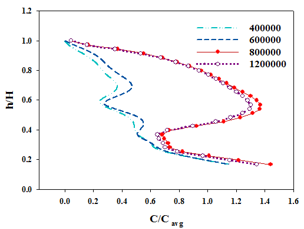Figure 2. Volume fraction of solid particles for different grid resolutions

## 3.png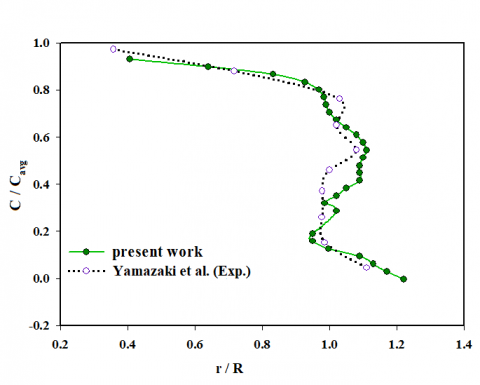Figure 3. Volume fraction of solid particles in a stirred tank

In the mixing processes, especially for the multi-phase mixing cases, the mode, the intensity and the time of mixing have significant effects on the mixing quality. To evaluate the quality of the mixing process, mixing properties of the mixer are usually measured by adding the tracer in the tank. The expected degree of homogeneity is obtained during the mixing time. Therefore, the mixing time is the time interval from the time of adding the tracer to that at which the specified degree of homogeneity is provided. The concentration history is calculated at five different locations in the tank as shown in Table 1. Two points (Pt-4 and Pt-5) are located above the impeller and two points (Pt-1 and Pt-2) are located below the impeller. The tracer concentration for each location is calculated and reported at the mixing time at which the tracer concentration lies within ±5 % of the specified concentration. Figure 4 illustrates dimensionless concentration of solid particles as a function of time for different mentioned locations for N=16.7 rps. Effective mixing time is the maximum time that is obtained from different locations.

It is known that as the rotational speed of the impeller increases, the volume fraction of solid particles in the upper part of the tank is negligible for high solid particle concentrations (≥10 %) . It can be concluded that the mixing time increases with the concentration of solid particles. Kasat et al.  revealed that the cloud height is a function of the impeller rotational speed. The cloud height is the distance of highest average volume fraction point from the bottom of the tank. Figure 5 shows the volume fraction of solid particles at mid baffle plane for three different impeller rotational speeds, N=5, 10, and 16.7 rps. Particle Reynolds number is defined based on the slip velocity, $Re_p=ρ_α d_p u ⃗_slip/μ_α$ . Since the particle drag coefficient is calculated by the equation:

$C_D=\frac{24}{Re_p}(1+0.2Re_p^{0.63})$ (10)

Table 1. Tracer locations

 x y z Pt-1 -0.006 0.07 0.1 Pt-2 0.02 0.04 0.03 Pt-3 0.08 0.08 0.055 Pt-4 0.08 0.1 0.18 Pt-5 0.05 0.01 0.255

## 4.png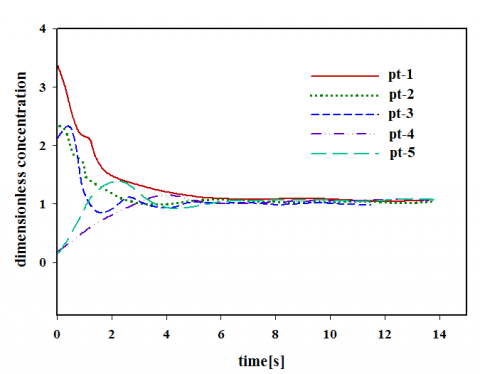Figure 4. Mixing time in terms of dimensionless tracer concentration profile for N=16.7 rps

Particle Reynolds number affects on particle drag coefficient. As the slip velocity increases, particle Reynolds number enhances. In other words, the turbulence increases the particle drag coefficient. At low impeller rotational speeds, the drag force on suspended particles decreases and all the energy of impeller is used to circulate the fluid. Hence, the mixing time decreases with the impeller rotational speed.

Impeller rotational speeds of 5, 10, and 16.7 rps lead to the Reynolds numbers equal to ,5*104 , 105 and 16.7*104, respectively. Drag coefficient correspond to these Reynolds numbers are 3.715, 3.748, and 3.77, respectively.

It should be pointed out that different values of rotational speed are selected according to the simulations of Kasat et al. . It can be seen that predicted mixing time decreases with the impeller rotational speed. Present simulations confirm the results of Kasat et al. and Galleti et al. who showed that only a single vortex is generated at low impeller rotational speed (≤5 rps) [4, 17]. As the impeller rotational speed increases, single vortex structure is converted to two vortices structure. Actually, the outward thrust of the impeller creates an outward jet. As the impeller rotational speed increases, the jet becomes stronger. The jet does not reach the wall of the tank at N=5 rps, but it approaches the wall and strikes it at higher impeller rotational speeds (N=10 and 16.7 rps). The jet is strong in the vicinity of the impeller blade. As the jet moves towards the wall, its momentum decreases. This causes that the jet splits into two axial flow results in the generation of a counterclockwise velocity field in the region above and a clockwise velocity field in the region below the impeller plane.

## 51.pngN = 5 rps

## 52.png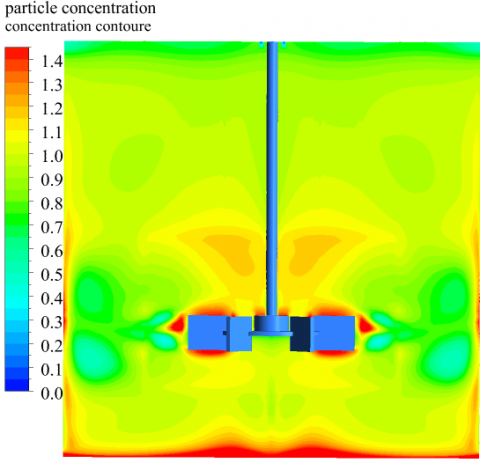N=10 rps

## 53.pngN=16.7 rps

Figure 5. Solid particle concentration contours for different impeller rotational speed

At impeller speed N=5 rps, a thin layer of particles is observed at the bottom of the tank and hence the particle concentration is low at z/H˂˂1. As the rotational speed of the impeller increases, the concentration of particles increases at the bottom of the tank and around the impeller surface. At N=16.7 rps, the aggregation of solid particles is more than that at N=5 and 10 rps. At this rotational speed, the generated vortices at the top and bottom of the tank become stronger results in stronger particle transporting at top and bottom of the tank. Therefore, concentration of solid particles decreases at the center of the vortices. In other words, the aggregation of solid particles at the points 4 and 5 enhances at high Reynolds numbers (high impeller rotational speeds). Figure 5 demonstrates that the concentration of suspended particles increases above the impeller by increasing the Reynolds number. One can predicts that a clear layer (a layer in which the concentration of solid particles is negligible) is generate around the impeller at very high impeller velocities.

In Figure 6, the concentration distribution of solid particles is plotted in two horizontal and vertical directions. It is concluded from the figure that concentration of solid particles is more uniform at higher speeds. As the impeller rotational speed increases, solid particles start suspending off the bottom of the tank leads to generation of two vortices above and below the impeller. These vortices increase the transfer of mass in these regions results in more uniformity of the flow.

The radial profiles of the average radial velocities is shown in figures 7a at different values of z/H. Four axial cross sections are considered: z/H=0.075 (close to the bottom of the tank), 0.25 (precisely below the impeller), 0.33 (at the impeller plane) and 0.62 (between the impeller surface and the top of the tank). As previously explained, two vortices are created above and below the impeller leads to a negative radial velocity for solid particles at z/H=0.075. As expected, the radial velocity on impeller surface is higher than that in other axial positions.

The maximum radial velocity at the impeller plane is 22 % of the impeller tip speed which is significantly different from the single-phase fluid flow in a similarly sized reactor  (they concluded that the maximum axial velocity is 48 % of the impeller tip speed). This is due to higher volume fraction of solid particles in the present simulations. On the other hand, the impeller radial velocity increases with increasing the particle concentration. It is observed from figure 8b that the radial velocity for different axial positions has a similar trend. The graphs are plotted in three axial positions at r/R = 0.33, 0.5, and 0.75. The redial velocity is identity for the positions of r/R=0.33 and 0.75 and is larger for the position of r/R=0.5. Similar behavior has been reported for the distribution of solid particles in an unbaffled stirred tank . The authors showed that this is due to the effect of different boundary conditions imposed at the bottom (no-slip boundary condition) and at the top (free-slip boundary condition).

3.2 Eulerian-Lagrangian approach

In the previous section, the results of the Eulerian-Eulerian model were presented. In this section, Eulerian-Lagrangian model is employed to study the distribution of solid particles in a stirred tank and also to compare the results of these models. In the Eulerian-Eulerian model, the governing equations are solved for two liquid and solid phases on an Eulerian grid while two phases are assumed to be continues. In the Eulerian-Lagrangian approach, the position of particles (or particle clusters) is tracked individually as they move through the inner region of the stirred tank. It should be noted that continues fluid phase is also applied to the Eulerian-Lagrangian approach. There are two methods describe the Eulerian-Lagrangian approach: point-particle and resolved-particle methods . The difference between these two methods is that the flow around the particle is not resolved in the point-particle one. The interaction between two solid and liquid phases is taken into account in the resolved-particle method. Here, the point-particle method is employed for simulating solid-liquid suspensions in the tank due to high computational cost of resolve-particle method.

## 61.png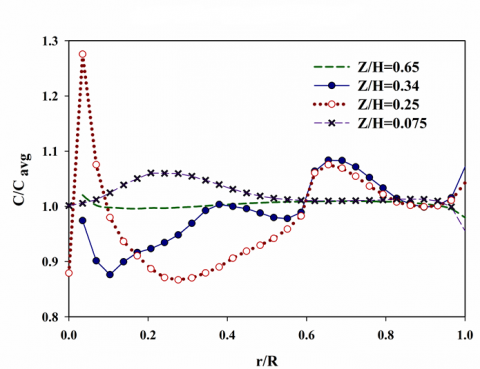N=5 rps

## 62.png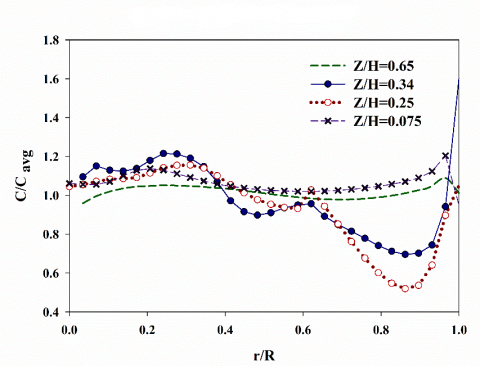N=10 rps

## 63.png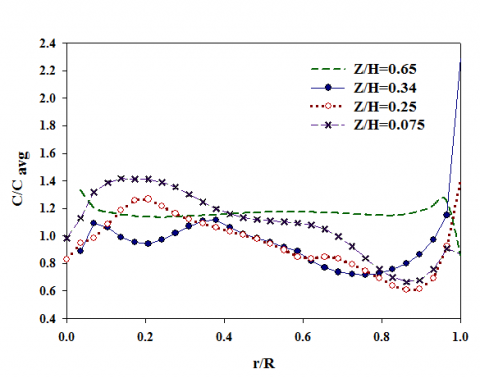N=16.7 rps

Figure 6. Volume fraction of solid particles for different impeller rotational speeds

Solid hold-up distribution obtained from two above approaches is shown in figure 8 in comparison with the results of Yamazaki et al. . Since a dense solid-liquid system is simulated in the present work, the point-particle method does not predict the values of the volume fraction of solid particles especially around the impeller blade. This is due to that this method employs the single-particle correlations to calculate the force acting on the particles . Therefore, this method is more appropriate for dilute solid-liquid suspensions. Also, point-particle method does not capture efficiently the interaction between the solid and liquid interface. As a result, the method concludes generation of different types of vortices above and below the impeller in comparison with the Eulerian-Lagrangian approach (Figure 9).

## 71.png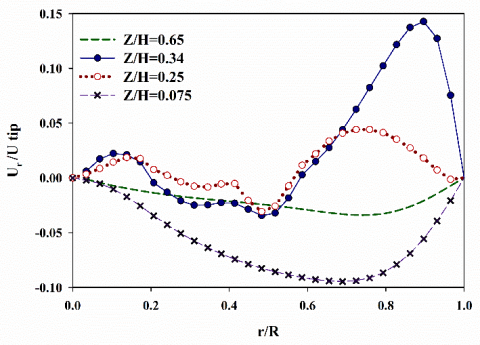(a)

## 72.png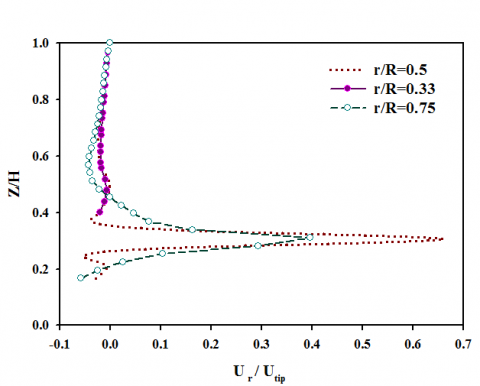(b)

Figure 7. Non-dimensional flow velocity in (a) the axial and (b)radial ditection

## 8.png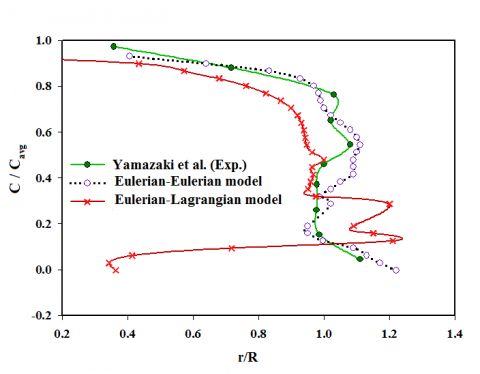Figure 8. Volume fraction of solid particles, N=15 rps

## 91.png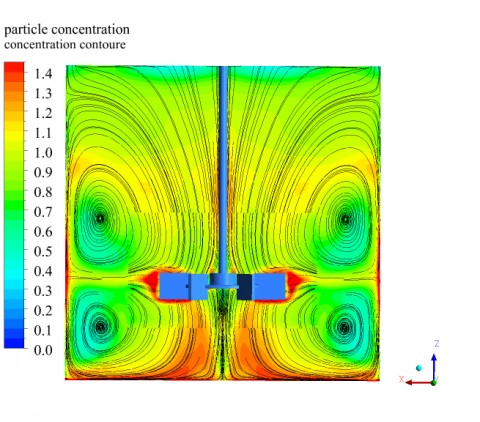(a)

## 92.png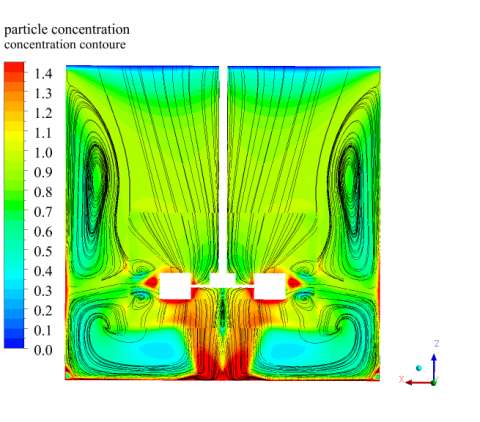(b)

Figure 9. Comparison of streamlines obtained from (a) Eulerian-Eulerian and (b) Eulerian-Lagrangian models, for N=16.7 rps

It should be pointed out that the study of microstructure of binary collision between solid particles or deformable drops (bubbles) is very important to predict the behaviour of their suspensions. For more details refer to [18-26].

4. Conclusions

Numerical simulation of the motion of solid particles in a baffled stirred tank was presented. The turbulent flow regime is the nature of the unsteady flows. The two-phase flow model along with the standard k-ε turbulence model were used to simulate the suspension of solid-liquid system. A multiple reference frames method (MRFs) was used to simulate the impeller rotation in the tank. The results were obtained by employing Eulerian-Eulerian and Eulerian-Lagrangian approaches. It was found that as the impeller rotational speed increases, the time of mixing increases. The outward thrust of the impeller creates an outward jet. The jet does not reach the wall of the tank at low impeller rotational speeds, but it approaches the wall and strikes it at high impeller rotational speeds. The results showed that the Eulerian-Lagrangian approach (the point-particle method) cannot describe the liquid-phase mixing process for a dense solid-liquid system.

Many investigations have been performed to evaluate the mixing efficiency in microfluidic devices, for example .

Nomenclature
 CD Drag coefficient dp Particle diameter (m) g Acceleration due to the gravity ( m/s2) H Diameter of the reactor (m) k Turbulent kinetic energy (J) L Height of the reactor (m) N Angular velocity (rps) P Pressure (Pa) r Radial direction (m) Re Reynolds number S Source term (J) t Time (s) v Velocity (m/s) x, y, z Spatial coordinates (m) Greek letters μ Viscosity (Pa.s) ρ Density ( kg/m3 ) $φ_s$ Solid particles volume fraction $τ ̿$ Shear stress tensor (Pa) ε Dissipation rate Subscripts p Particle r Radial s Solid slip Slip f Fluid
References

 Yamazaki H, Tojo K, Miyanami K. (1986). Concentration profiles of solids suspended in a stirred tank. Powder Technology 48: 205-216. http://dx.doi.org/10.1016/0032-5910(86)80043-2

 Gosman AD, Lekakou C, Politis S. (1992). Multidimensional modeling of turbulent two‐phase flows in stirred vessels. AIChE Journal 38(12): 1946-1956. http://dx.doi.org/10.1002/aic.690381210 ‏

 Sbrizzai F, Lavezzo V, Verzicco R, Campolo M, Soldati A. (2016). Direct numerical simulation of turbulent particle dispersion in an unbaffled stirred-tank reactor. Chemical Engineering Science 61: 2843-2851. http://dx.doi.org/10.1016/j.ces.2005.10.073

 Kasat GR, Khopkar AR, Ranade VV, Pandit AB. (2008). CFD simulation of liquid-phase mixing in solid–liquid stirred reactor. Chemical Engineering Science 63(15): 3877-3885. http://dx.doi.org/10.1016/j.ces.2008.04.018 ‏

 Radl S, Sundaresan S. (2014) A drag model for filtered Euler–Lagrange simulations of clustered gas–particle suspensions. Chemical Engineering Science 117: 416-425. http://dx.doi.org/10.1016/j.ces.2014.07.011

 Montante G, Magelli F. (2005). Modelling of solids distribution in stirred tanks: Analysis of simulation strategies and comparison with experimental data. International Journal of Computational Fluid Dynamics 19(3): 253-262. https://doi.org/10.1080/10618560500081795

 Bashiri H, Alizadeh E, Bertrand F, Chaouki J. (2016). Investigation of turbulent fluid flows in stirred tanks using a non-intrusive particle tracking technique. Chemical Engineering Science 140: 233–251. http://dx.doi.org/10.1016/j.ces.2015.10.005

 Lassaigne M, Blais B, Fradette L, Bertrand F. (2016). Experimental investigation of the mixing of viscous liquids and non-dilute concentrations of particles in a stirred tank. Chem. Eng. Res. Des. 108: 55–68. http://dx.doi.org/10.1016/j.cherd.2016.01.005

 Wadnerkar D, Tade MO, Pareek VK, Utikar RP. (2016) CFD simulation of solid–liquid stirred tanks for low to dense solid loading systems. Advanced Powder Technology 29: 16–33. http://dx.doi.org/10.1016/j.partic.2016.01.012

 Tamburini A, Cipollina A, Micale G, Brucato A, Ciofalo M. (2011). CFD simulations of dense solid-liquid suspensions in baffled stirred tanks: Prediction of suspension curves. Chemical Engineering Journal 178: 324-341. http://dx.doi.org/10.1016/j.cej.2011.10.016

 Oyegbile B, Akdogan G, Karimi M. (2018). Experimental and CFD studies of the hydrodynamics in wet agglomeration process. Chem. Engineering 2(3): 32. https://doi.org/10.3390/ chemengineering2030032

 Liu XQ, Yue S, Lu LY, Gao W, Li JL. (2018). Numerical simulations of a gas-solid two-phase impinging stream reactor with dynamic inlet flow, Energies 11(7): 1913. http://doi.org/10.3390/en11071913

 Guha D, Ramachandran PA, Dudukovic MP. (2007). Flow field of suspended solids in a stirred tank reactor by Lagrangian tracking. Chemical Engineering Science 62(22): 6143-6154. http://dx.doi.org/10.1016/j.ces.2007.06.033

 Mishra P, Ein-Mozaffari F. (2017) Using computational fluid dynamics to analyze the performance of the Maxblend impeller in solid-liquid mixing operations. International Journal of Multiphase Flow 91: 194-207. http://dx.doi.org/10.1016/j.ijmultiphaseflow.2017.01.009

 Rammohan AR, Kemoun A, Al-Dahhan MH, Dudukovic MP. (2001). Characterization of single phase flows in stirred tanks via computer automated radioactive particle tracking (CARPT). Chemical Engineering Research and Design 79(8): 831-844. http://dx.doi.org/10.1205/02638760152721343

 Li Z, Derksen JJ, Gao Z. (2015). Models and applications for simulating turbulent solid-liquid suspensions in stirred tanks. Journal of Chemical Engineering of Japan 48(5): 329-336. http://dx.doi.org/10.1252/jcej.14we056

 Galleti C, Brunazzi E, Yianneskis M, Paglianti A. (2003). Spectral and wavelet analysis of the flow pattern transition with impeller clearance variations in a stirred vessel. Chemical Engineering Science 58: 3859-3875. http://dx.doi.org/10.1016/S0009-2509(03)00230-6

 Goodarzi Z, Ahmadi Nadooshan A, Bayareh M. (2018). Numerical investigation of off-centre binary collision of droplets in a horizontal channel. Journal of the Brazilian Society of Mechanical Sciences and Engineering 40: 1-10. http://dx.doi.org/10.1007/s40430-018-1075-y

 Armandoost P, Bayareh M, Ahmadi Nadooshan A. (2018). Study of the motion of a spheroidal drop in a linear shear flow. Journal of Mechanical Science and Technology 32: 2059-2067. http://dx.doi.org/10.1007/s12206-018-0415-2

 Bayareh M, Mortazavi S. (2011). Effect of density ratio on the hydrodynamic interaction between two drops in simple shear flow. Iranian Journal of Science and Technology 35: 441-452. http://doi.org/10.22099/ijstm.2011.900

 Bayareh M, Mortazavi S. (2013). Equilibrium position of a buoyant drop in Couette and Poiseuille flows at finite Reynolds numbers. Journal of Mechanics 29: 53-58. http://dx.doi.org/10.1017/jmech.2012.109

 Bayareh M, Mortazavi S. (2009). Geometry effects on the interaction of two equal-sized drops in simple shear flow at finite Reynolds numbers. Eng. Sci. 63: 379-388. http://doi.org/10.2495/MPF090321

 Bayareh M, Mortazavi S. (2010) Migration of a drop in simple shear flow at finite Reynolds numbers: Size and viscosity ratio effects. Proceeding of International Conference on Mechanical, Industriel and Manufacturing Engineering (ICMIME), Cape Town, South Africa, pp. 287-293.

 Mohammadi Masiri S, Bayareh M, Ahmadi Nadooshan A. (2019) Pairwise intercation of drops in shear-thinning inelastic fluids. Korea-Australia Rheology Journal 31(1): 25-34. https://doi.org/10.1007/s13367-019-0003-8

 Bayareh M, Nourbakhsh A. (2018) Study of the bubble motion in a compound couette-Poiseuille flow: Effect of the pressure gradient. Advances in Modelling and Analysis A 55(1): 11-19. https://doi.org/10.18280/ama_a.550102

 Bayareh M. Dabiri S. Ardekani AM. (2016) Interaction between two drops ascending in a linearly stratified fluid. European Journal of Mechanics-B/Fluids 60: 127-136. http://dx.doi.org/10.1016/j.euromechflu.2016.07.002

 Usefian A, Bayareh M, Ahmadi Nadooshan A. (2018) Rapid mixing of Newtonian and non-Newtonian fluids in a three-dimensional micro-mixer using non-uniform magnetic field. Journal of Heat and Mass Transfer Research. http://doi.org/10.22075/jhmtr.2018.15611.1218